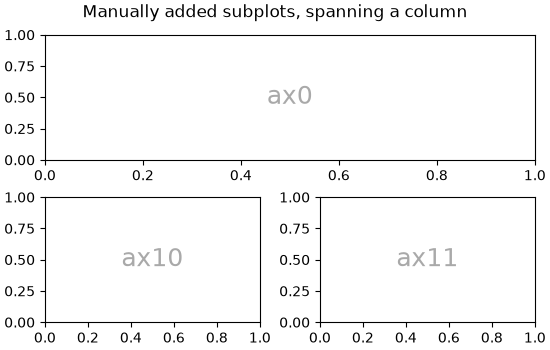# Arranging multiple Axes in a Figure#

Often more than one Axes is wanted on a figure at a time, usually organized into a regular grid. Matplotlib has a variety of tools for working with grids of Axes that have evolved over the history of the library. Here we will discuss the tools we think users should use most often, the tools that underpin how Axes are organized, and mention some of the older tools.

Note

Matplotlib uses Axes to refer to the drawing area that contains data, x- and y-axis, ticks, labels, title, etc. See Parts of a Figure for more details. Another term that is often used is "subplot", which refers to an Axes that is in a grid with other Axes objects.

## Overview#

### Create grid-shaped combinations of Axes#

`subplots`

The primary function used to create figures and a grid of Axes. It creates and places all Axes on the figure at once, and returns an object array with handles for the Axes in the grid. See `Figure.subplots`.

or

`subplot_mosaic`

A simple way to create figures and a grid of Axes, with the added flexibility that Axes can also span rows or columns. The Axes are returned in a labelled dictionary instead of an array. See also `Figure.subplot_mosaic` and Complex and semantic figure composition.

Sometimes it is natural to have more than one distinct group of Axes grids, in which case Matplotlib has the concept of `SubFigure`:

`SubFigure`

A virtual figure within a figure.

### Underlying tools#

Underlying these are the concept of a `GridSpec` and a `SubplotSpec`:

`GridSpec`

Specifies the geometry of the grid that a subplot will be placed. The number of rows and number of columns of the grid need to be set. Optionally, the subplot layout parameters (e.g., left, right, etc.) can be tuned.

`SubplotSpec`

Specifies the location of the subplot in the given `GridSpec`.

### Adding single Axes at a time#

The above functions create all Axes in a single function call. It is also possible to add Axes one at a time, and this was originally how Matplotlib used to work. Doing so is generally less elegant and flexible, though sometimes useful for interactive work or to place an Axes in a custom location:

`add_axes`

Adds a single axes at a location specified by `[left, bottom, width, height]` in fractions of figure width or height.

`subplot` or `Figure.add_subplot`

Adds a single subplot on a figure, with 1-based indexing (inherited from Matlab). Columns and rows can be spanned by specifying a range of grid cells.

`subplot2grid`

Similar to `pyplot.subplot`, but uses 0-based indexing and two-d python slicing to choose cells.

## High-level methods for making grids#

### Basic 2x2 grid#

We can create a basic 2-by-2 grid of Axes using `subplots`. It returns a `Figure` instance and an array of `Axes` objects. The Axes objects can be used to access methods to place artists on the Axes; here we use `annotate`, but other examples could be `plot`, `pcolormesh`, etc.

```import matplotlib.pyplot as plt
import numpy as np

fig, axs = plt.subplots(ncols=2, nrows=2, figsize=(5.5, 3.5),
constrained_layout=True)
# add an artist, in this case a nice label in the middle...
for row in range(2):
for col in range(2):
axs[row, col].annotate(f'axs[{row}, {col}]', (0.5, 0.5),
transform=axs[row, col].transAxes,
ha='center', va='center', fontsize=18,
color='darkgrey')
fig.suptitle('plt.subplots()')
```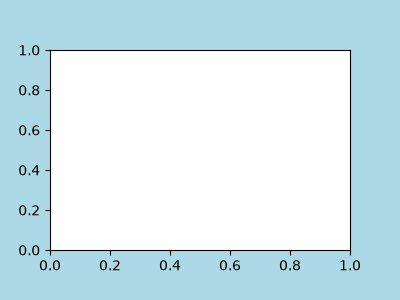Out:

```Text(0.5, 0.9880942857142857, 'plt.subplots()')
```

We will annotate a lot of Axes, so lets encapsulate the annotation, rather than having that large piece of annotation code every time we need it:

```def annotate_axes(ax, text, fontsize=18):
ax.text(0.5, 0.5, text, transform=ax.transAxes,
ha="center", va="center", fontsize=fontsize, color="darkgrey")
```

The same effect can be achieved with `subplot_mosaic`, but the return type is a dictionary instead of an array, where the user can give the keys useful meanings. Here we provide two lists, each list representing a row, and each element in the list a key representing the column.

```fig, axd = plt.subplot_mosaic([['upper left', 'upper right'],
['lower left', 'lower right']],
figsize=(5.5, 3.5), constrained_layout=True)
for k in axd:
annotate_axes(axd[k], f'axd["{k}"]', fontsize=14)
fig.suptitle('plt.subplot_mosaic()')
```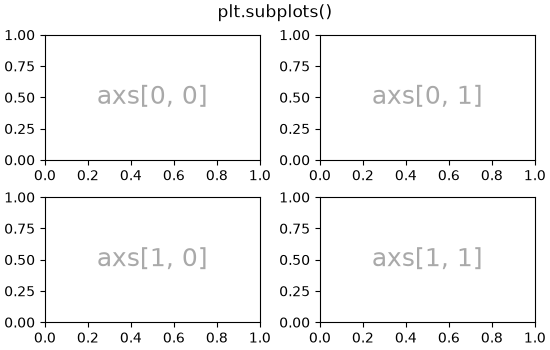Out:

```Text(0.5, 0.9880942857142857, 'plt.subplot_mosaic()')
```

### Axes spanning rows or columns in a grid#

Sometimes we want Axes to span rows or columns of the grid. There are actually multiple ways to accomplish this, but the most convenient is probably to use `subplot_mosaic` by repeating one of the keys:

```fig, axd = plt.subplot_mosaic([['upper left', 'right'],
['lower left', 'right']],
figsize=(5.5, 3.5), constrained_layout=True)
for k in axd:
annotate_axes(axd[k], f'axd["{k}"]', fontsize=14)
fig.suptitle('plt.subplot_mosaic()')
```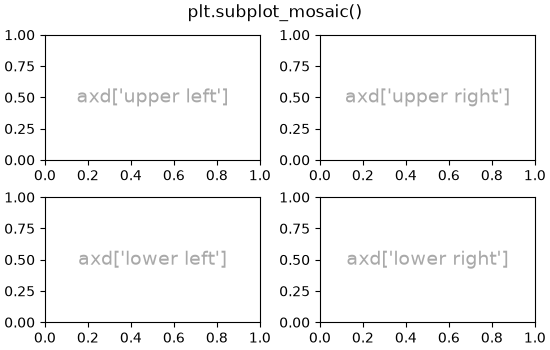Out:

```Text(0.5, 0.9880942857142857, 'plt.subplot_mosaic()')
```

See below for the description of how to do the same thing using `GridSpec` or `subplot2grid`.

### Variable widths or heights in a grid#

Both `subplots` and `subplot_mosaic` allow the rows in the grid to be different heights, and the columns to be different widths using the gridspec_kw keyword argument. Spacing parameters accepted by `GridSpec` can be passed to `subplots` and `subplot_mosaic`:

```gs_kw = dict(width_ratios=[1.4, 1], height_ratios=[1, 2])
fig, axd = plt.subplot_mosaic([['upper left', 'right'],
['lower left', 'right']],
gridspec_kw=gs_kw, figsize=(5.5, 3.5),
constrained_layout=True)
for k in axd:
annotate_axes(axd[k], f'axd["{k}"]', fontsize=14)
fig.suptitle('plt.subplot_mosaic()')
```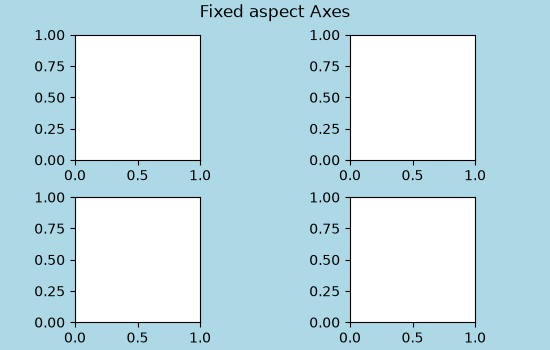Out:

```Text(0.5, 0.9880942857142857, 'plt.subplot_mosaic()')
```

### Nested Axes layouts#

Sometimes it is helpful to have two or more grids of Axes that may not need to be related to one another. The most simple way to accomplish this is to use `Figure.subfigures`. Note that the subfigure layouts are independent, so the Axes spines in each subfigure are not necessarily aligned. See below for a more verbose way to achieve the same effect with `GridSpecFromSubplotSpec`.

```fig = plt.figure(constrained_layout=True)
subfigs = fig.subfigures(1, 2, wspace=0.07, width_ratios=[1.5, 1.])
axs0 = subfigs.subplots(2, 2)
subfigs.set_facecolor('0.9')
subfigs.suptitle('subfigs\nLeft side')
subfigs.supxlabel('xlabel for subfigs')

axs1 = subfigs.subplots(3, 1)
subfigs.suptitle('subfigs')
subfigs.supylabel('ylabel for subfigs')
```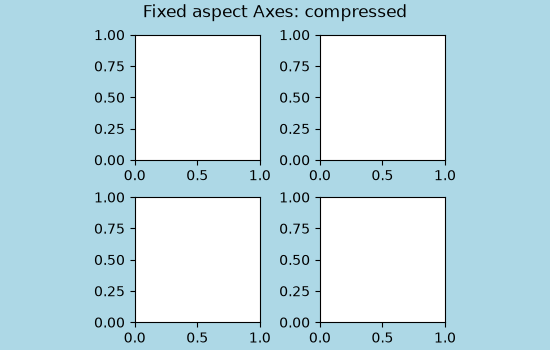Out:

```Text(0.016867713730569944, 0.5, 'ylabel for subfigs')
```

It is also possible to nest Axes using `subplot_mosaic` using nested lists. This method does not use subfigures, like above, so lacks the ability to add per-subfigure `suptitle` and `supxlabel`, etc. Rather it is a convenience wrapper around the `subgridspec` method described below.

```inner = [['innerA'],
['innerB']]
outer = [['upper left',  inner],
['lower left', 'lower right']]

fig, axd = plt.subplot_mosaic(outer, constrained_layout=True)
for k in axd:
annotate_axes(axd[k], f'axd["{k}"]')
```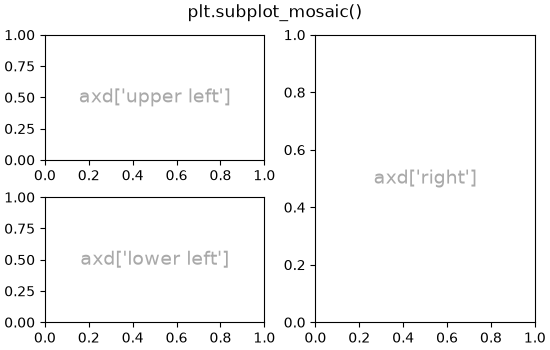## Low-level and advanced grid methods#

Internally, the arrangement of a grid of Axes is controlled by creating instances of `GridSpec` and `SubplotSpec`. GridSpec defines a (possibly non-uniform) grid of cells. Indexing into the GridSpec returns a SubplotSpec that covers one or more grid cells, and can be used to specify the location of an Axes.

The following examples show how to use low-level methods to arrange Axes using GridSpec objects.

### Basic 2x2 grid#

We can accopmplish a 2x2 grid in the same manner as `plt.subplots(2, 2)`:

```fig = plt.figure(figsize=(5.5, 3.5), constrained_layout=True)

annotate_axes(ax0, 'ax0')

annotate_axes(ax1, 'ax1')

annotate_axes(ax2, 'ax2')

annotate_axes(ax3, 'ax3')

```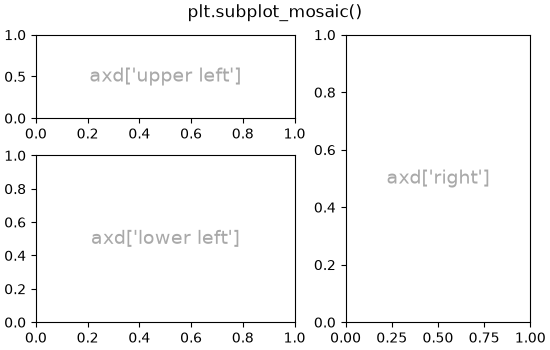Out:

```Text(0.5, 0.9880942857142857, 'Manually added subplots using add_gridspec')
```

### Axes spanning rows or grids in a grid#

We can index the spec array using NumPy slice syntax and the new Axes will span the slice. This would be the same as `fig, axd = plt.subplot_mosaic([['ax0', 'ax0'], ['ax1', 'ax2']], ...)`:

```fig = plt.figure(figsize=(5.5, 3.5), constrained_layout=True)

annotate_axes(ax0, 'ax0')

annotate_axes(ax10, 'ax10')

annotate_axes(ax11, 'ax11')

fig.suptitle('Manually added subplots, spanning a column')
```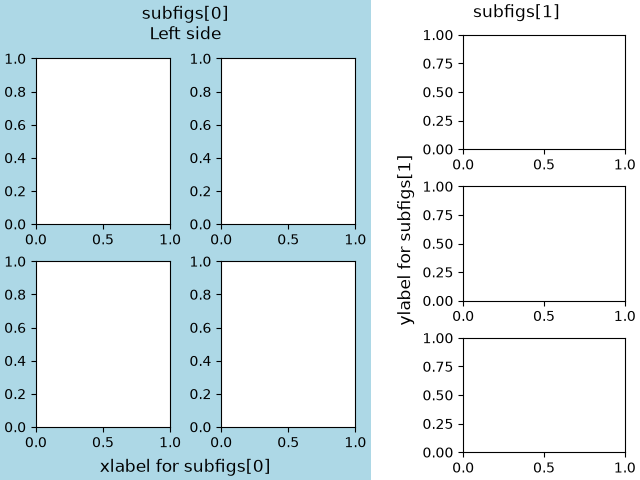Out:

```Text(0.5, 0.9880942857142857, 'Manually added subplots, spanning a column')
```

### Manual adjustments to a GridSpec layout#

When a GridSpec is explicitly used, you can adjust the layout parameters of subplots that are created from the GridSpec. Note this option is not compatible with `constrained_layout` or `Figure.tight_layout` which both ignore left and right and adjust subplot sizes to fill the figure. Usually such manual placement requires iterations to make the Axes tick labels not overlap the Axes.

These spacing parameters can also be passed to `subplots` and `subplot_mosaic` as the gridspec_kw argument.

```fig = plt.figure(constrained_layout=False, facecolor='0.9')
gs = fig.add_gridspec(nrows=3, ncols=3, left=0.05, right=0.75,
hspace=0.1, wspace=0.05)
annotate_axes(ax0, 'ax0')
annotate_axes(ax1, 'ax1')
annotate_axes(ax2, 'ax2')
fig.suptitle('Manual gridspec with right=0.75')
```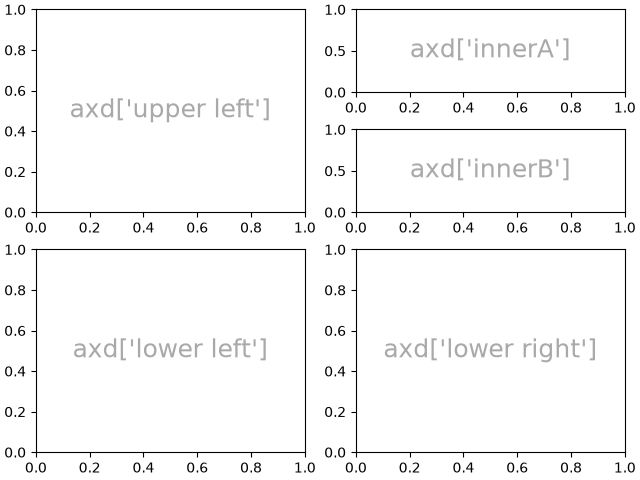Out:

```Text(0.5, 0.98, 'Manual gridspec with right=0.75')
```

### Nested layouts with SubplotSpec#

You can create nested layout similar to `subfigures` using `subgridspec`. Here the Axes spines are aligned.

Note this is also available from the more verbose `gridspec.GridSpecFromSubplotSpec`.

```fig = plt.figure(constrained_layout=True)

gs00 = gs0.subgridspec(2, 2)
gs01 = gs0.subgridspec(3, 1)

for a in range(2):
for b in range(2):
annotate_axes(ax, f'axLeft[{a}, {b}]', fontsize=10)
if a == 1 and b == 1:
ax.set_xlabel('xlabel')
for a in range(3):
annotate_axes(ax, f'axRight[{a}, {b}]')
if a == 2:
ax.set_ylabel('ylabel')

fig.suptitle('nested gridspecs')
```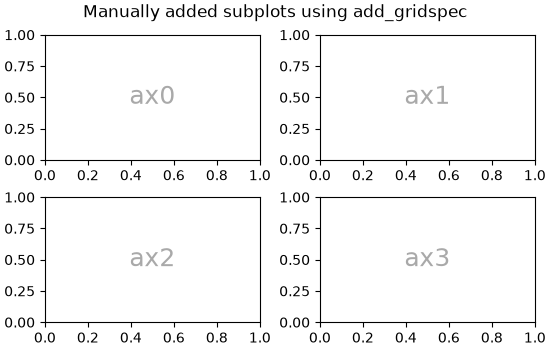Out:

```Text(0.5, 0.99131875, 'nested gridspecs')
```

Here's a more sophisticated example of nested GridSpec: We create an outer 4x4 grid with each cell containing an inner 3x3 grid of Axes. We outline the outer 4x4 grid by hiding appropriate spines in each of the inner 3x3 grids.

```def squiggle_xy(a, b, c, d, i=np.arange(0.0, 2*np.pi, 0.05)):
return np.sin(i*a)*np.cos(i*b), np.sin(i*c)*np.cos(i*d)

fig = plt.figure(figsize=(8, 8), constrained_layout=False)
outer_grid = fig.add_gridspec(4, 4, wspace=0, hspace=0)

for a in range(4):
for b in range(4):
# gridspec inside gridspec
inner_grid = outer_grid[a, b].subgridspec(3, 3, wspace=0, hspace=0)
axs = inner_grid.subplots()  # Create all subplots for the inner grid.
for (c, d), ax in np.ndenumerate(axs):
ax.plot(*squiggle_xy(a + 1, b + 1, c + 1, d + 1))
ax.set(xticks=[], yticks=[])

# show only the outside spines
for ax in fig.get_axes():
ss = ax.get_subplotspec()
ax.spines.top.set_visible(ss.is_first_row())
ax.spines.bottom.set_visible(ss.is_last_row())
ax.spines.left.set_visible(ss.is_first_col())
ax.spines.right.set_visible(ss.is_last_col())

plt.show()
```